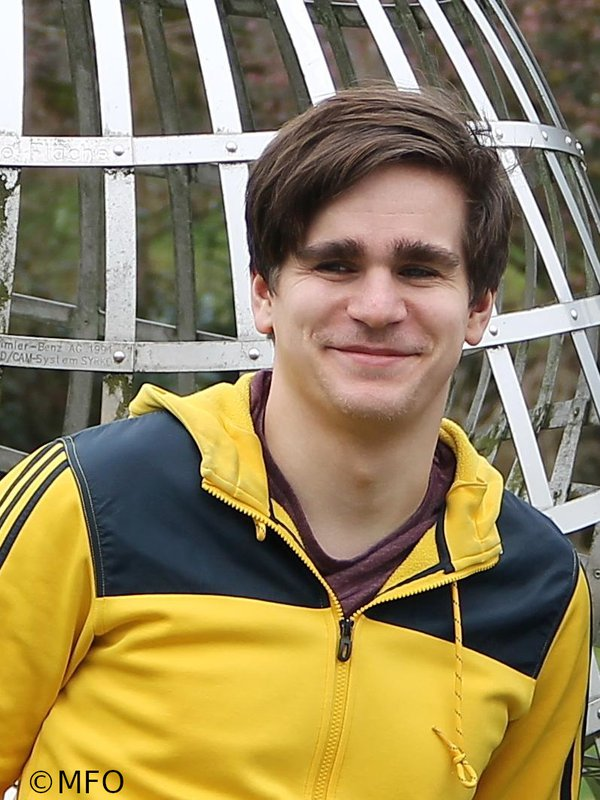Dr. Christoph Zimmer

Research Associate
Computational Mathematics
 Phone: +49 821 598-3932 Email: To show mail address please enable JavaScript! Room: 2316 (I) Visiting hours: by appointment Address: Universitätsstraße 12a, 86159 AugsburgPublications

  R. Altmann and C. Zimmer. Runge-Kutta methods for linear semi-explicit operator differential-algebraic equations. Math. Comp., Vol. 87, 2018, pp. 149–174.  A. Moses Badlyan and C. Zimmer. Operator-GENERIC formulation of thermodynamics of irreversible processes. ArXiv Preprint 1807.09822, 2018.  R. Altmann and C. Zimmer. On the smoothing property of linear delay partial differential equations. J. Math. Anal. Appl., Vol. 467, 2018, pp. 916–934.  R. Altmann and C. Zimmer. Time discretization schemes for hyperbolic systems on networks by ε-expansion. ArXiv Preprint 1810.04278, 2018 (also Oberwolfach Preprint 2019-03).  R. Altmann and C. Zimmer. Exponential integrators for semi-linear parabolic problems with linear constraints. In Progress in Differential-Algebraic Equations II, Springer, Cham, pp. 137–164, 2020.  R. Altmann and C. Zimmer. Singular perturbation results for linear partial differential-algebraic equations of hyperbolic type. ArXiv Preprint 2102.03177, 2021.  R. Altmann, B. Kovács, and C. Zimmer. Bulk-surface Lie splitting for parabolic problems with dynamic boundary conditions. ArXiv Preprint 2108.08147, 2021.

Theses

 [Th1] C. Zimmer. Adaptive Simulation eines Elastischen Pendels. Bachelor's thesis, TU Berlin, 2013. [Th2] C. Zimmer. Theorie und Numerik von Linearen Operator-Differentiell-Algebraischen Gleichungen mit Zeitverzögertem Term. Master's thesis, TU Berlin, 2015. [Th3] C. Zimmer. Temporal Discretization of Constrained Partial Differential Equations. Dissertation, TU Berlin, 2021